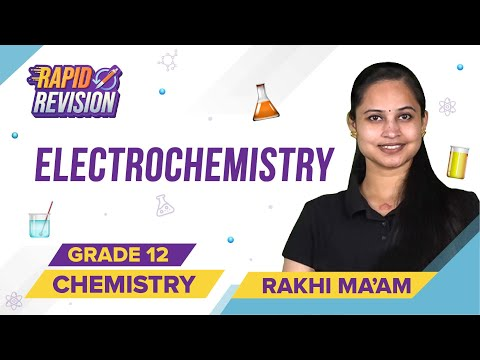Jet Set Go! All about Aeroplanes Jet Set Go! All about Aeroplanes

# Galvanic Cells Questions

Galvanic cells are the electrochemical cells in which the electrical energy is produced from suitable redox reactions. The Galvanic cells or the voltaic cells are named after the scientists who first used these cells for the energy conversion namely Luigi Galvani and Alessandro Volta. The galvanic cells convert the heat energy emitted from the redox reactions carried out within the same beaker into electrical energy.

 Definition: A Galvanic cell is a device that converts heat energy or chemical energy into electricity.

## Galvanic Cells Chemistry Questions with Solutions

Q1: What is the unit of equivalent conductance?

1. -1 cm-1
2. -1 cm-2
3. -1 cm2
4. None of the above

Explanation: Since Equivalent conductivity = Specific conductance x Volume

Hence, units of Equivalent conductivity = Ω-1 cm-1 x cm3 = Ω-1 cm2

Q2. In an electrochemical cell:

1. Potential energy decreases
2. Kinetic energy decreases
3. Chemical energy gets converted into electrochemical energy
4. Potential energy gets converted into electrical energy

Explanation: In an electrochemical cell the chemical energy gets converted into electrochemical energy

Q3. What happens when a lead storage battery gets discharged?

1. Sulphuric acid is consumed
4. SO2 gas is evolved

Explanation: When a lead storage battery gets discharged, acid is consumed releasing water.

Q4. A SHE has a zero potential because

1. H is the lightest element
2. H is easier to oxidise
3. An H-atom has only 1 electron
4. This electrode potential is assumed to be zero

Explanation: This is because SHE is taken as a reference electrode. Hence, SHE’s potential is assumed to be zero.

Q5. Write the Nernst Equation for a single electrode.

Answer: Let us assume a half-cell reaction: Mn+ + ne → M

The Nernst equation for this equation can be derived as:

EMn+/M = EoMn+/M – (2.303RT / nF) log([M] / [Mn+]) = EoMn+/M – (2.303RT / nF) log(1 / [Mn+])

Q6. Which electrodes are used in fuel cells?

Answer: Hydrogen electrode and oxygen electrode

Q7. What is overvoltage?

Answer: The voltage required for the deposition of metals on the cathode is just the same as the standard electrode potential. However, some electrochemical processes are kinetically too slow to be carried at the same voltage. Hence, some extra voltage is required other than the theoretical value of standard electrode potential to pace up these reactions. This extra required voltage is called the overvoltage.

Q8. Why does a cell stop working after some time?

Answer: As the electrochemical cell involves a set of oxidation-reduction reactions going on inside, the concentrations of the electrolytic solutions keep changing with time. The change in concentration of the electrolytes brings about a change in their respective potentials. Hence, when the electrode potential of the two cells become equal, the cell stops working.

Q9. Mention 2 advantages of a hydrogen-oxygen fuel cell over an ordinary cell.

Answer: The advantages of hydrogen-oxygen fuel cell over an ordinary cell are:

1. They do not cause any pollution
2. They have higher efficiency than the ordinary cell (60-70%).

Q10. State the major factor that will influence the electrode potential of the following cell:

Mg (s) | Mg2+ (aq) || Ag+ (aq) | Ag (s)

Answer: The major factors that will influence the potential of the given cell are temperature and the concentrations of the Mg2+ and Ag+ ions.

Q11. Why is Chromium used for coating Iron?

Answer: This is because chromium does not corrode easily and hence, forms a protective layer on iron which is a highly corrosive metal.

Q12. What is the electrolyte that is used in a dry cell?

Answer: The electrolyte used involves a paste of NH4Cl, MnO2 and carbon.

Q13. What is the EMF when the cell reactions attain an equilibrium?

Q14. What flows inside the internal circuits of the galvanic cells?

Q15. How can you determine whether the given electrolyte is a strong electrolyte or weak electrolyte?

Answer: If the aqueous solution of the given electrolyte conducts electricity to a large extent, then, it must be a strong electrolyte. However, if the aqueous solution of the given electrolyte conducts electricity to a small extent, then it must be a weak electrolyte.

## Practise Questions on Galvanic Cells

Q1. The conductivity cell containing a 0.001 M KCl solution at 298 K has a resistance of 1500 Ω. What is the cell constant?

The conductivity of the 0.001 M KCl solution at 298 K is 0.146 x 10-3 S cm-1.

Q2. How much electricity (Faraday) is required to produce 20 g of calcium from molten calcium chloride (CaCl2)?

Q3. What amount of electricity (in Coulombs) is required to reduce 1 mole of Cr2O72- in the following reaction?

Cr2O72- + 14H+ + 6e → 2Cr3+ + 7H2O

Q4. Suggest any two materials that can be used in place of hydrogen in a fuel cell.

Q5. Why does the conductivity of a solution decrease with dilution?

Click the PDF to check the answers for Practice Questions.

## Electrochemistry## Galvanic Cells